# Fixed Field Analysis

## Overview

The Fixed Field Analysis provide the user with the default Analysis model that is custom built into ValuePRO. The fields, areas and calculations on this model are fixed and cannot be modified, with the exception of the Ancillary Items section.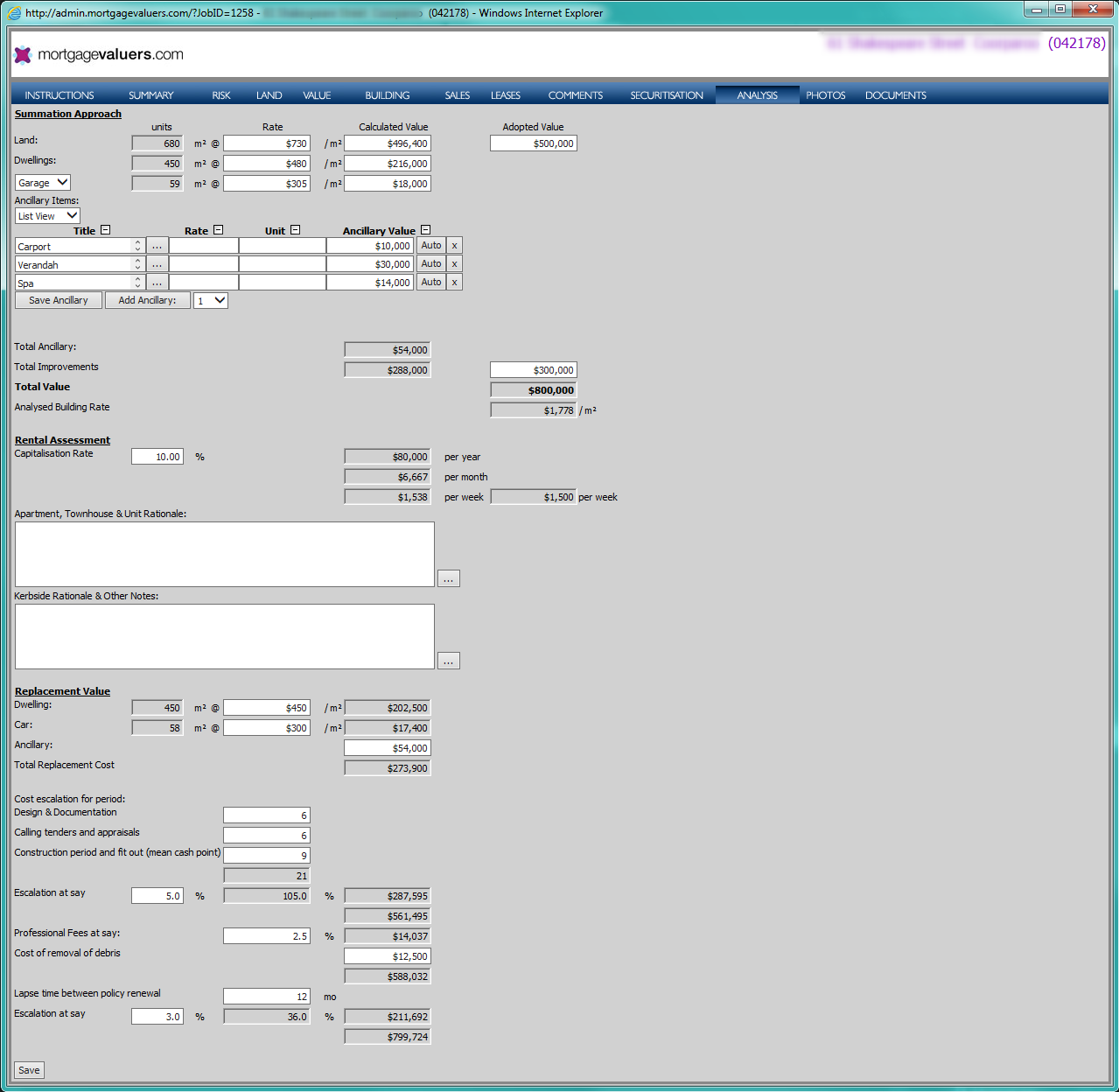## Summation Approach

The Summation Approach section provides the user with a set of fields to calculate the Market Value amount. All area values are sourced directly from the Job card and allow the user to enter a rate per m2 to calculate a value.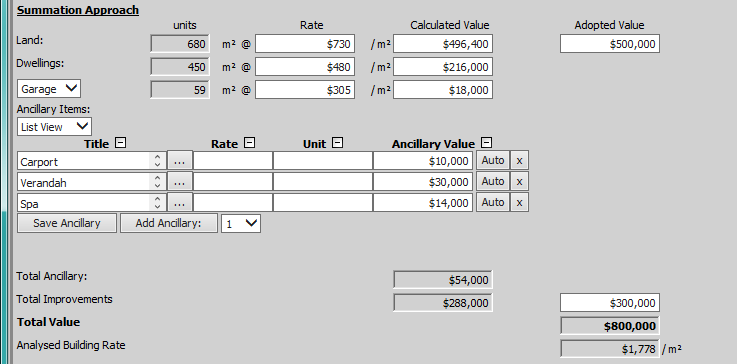### Land

The Land row provides the user with the following fields:

1. Units - The Units value is sourced from the Site Area field and this field is not editable by the user. In order to change this value, the user will need to locate the Site Area field on the Job card and update the value in that field.
2. Rate - The user can enter a rate per square metre value in this field. This value will be saved into the LandRate field.
3. Calculated Value - The Calculated Value is the value of: Units value x Rate value. Alternatively, the user can enter an amount into the Calculated Value field and ValuePRO will divide the Calculated Value amount by the Units value and fill in the Rate field for the user.
4. Adopted Value - The user can use the Calculated Value to determine a value that they wish to enter into the Adopted Value field, which would be used in the Total Value and Analysed Building Rate calculation fields. For example, the user may want to round up the Calculated Value to the nearest hundred or thousand to be used in the calculations.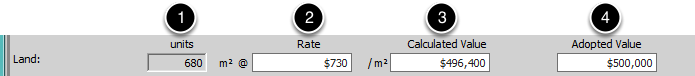### Dwellings

By default, this row of calculation fields is called Dwellings, however the label can be changed to any text by modifying the Analysis Dwelling Row Label setting.

The Dwellings row provides the user with the following fields:

1. Units - The Units value is sourced from the Living Areas field and this field is not editable by the user. In order to change this value, the user will need to locate the Living Areas field on the Job card and update the value in that field.
2. Rate - The user can enter a rate per square metre value in this field. This value will be saved into the MainBuildingRate field.
3. Calculated Value - The Calculated Value is the value of: Units value x Rate value. Alternatively, the user can enter an amount into the Calculated Value field and ValuePRO will divide the Calculated Value amount by the Units value and fill in the Rate field for the user.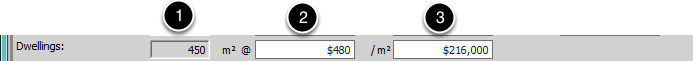### Car Accommodation

If the Display Analysis Car Label as Select List setting is turned off, the label for this row will be Car (4). However, if the setting is turned on, a select list will be available with two options for selection: Garage and Carport. The select list defaults to Garage.

The Car Accomodation row provides the user with the following fields:

1. Units - The Units value is sourced from the Car Areas field and this field is not editable by the user. In order to change this value, the user will need to locate the Car Areas field on the Job card and update the value in that field.
2. Rate - The user can enter a rate per square metre value in this field. This value will be saved into the CarRate field.
3. Calculated Value - The Calculated Value is the value of: Units value x Rate value. Alternatively, the user can enter an amount into the Calculated Value field and ValuePRO will divide the Calculated Value amount by the Units value and fill in the Rate field for the user.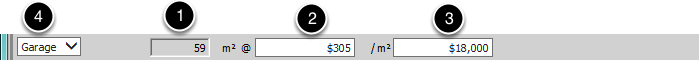### Ancillary Items

By default, ValuePRO includes an Ancillary Sub Object (1) on the DET which is then displayed on the Analysis tab. This allows the user to enter individual ancillary items and specify a title and a value for each item. The value for each individual ancillary item is then totaled and added to the Total Ancillary field (2), which is not editable.

Alternatively, if the Ancillary Sub Object is not present on the DET, the user will instead be presented with the Total Ancillary field (2) made editable to allow the user to enter a total value.

The Total Ancillary value is saved into the AncillaryValue field.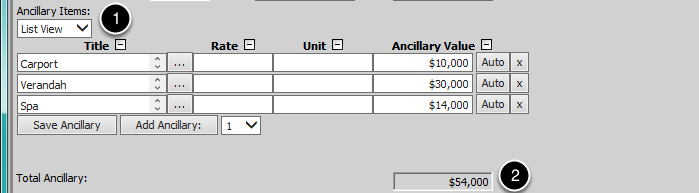### Total Improvements

The Total Improvements field in the Calculated Value column (1) contains the total value calculated as follows:

Dwellings Calculated Value + Car Accommodation Calculated Value + Total Ancillary Value = Total Improvements value

This field is not editable.

The Total Improvements field in the Adopted Value column (2) can be used to enter value determined from the Total Improvements Calculated Value field. For example, the user may want to round up the Calculated Value to the nearest hundred or thousand.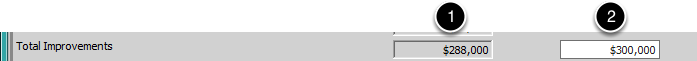### Total Value

The Total Value field contains the total value calculated as follows:

This field is not editable.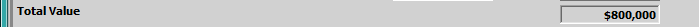### Analysed Land and Building Rate

The Analysed Building Rate field contains the calculated value amount per square metre for the entire property. The value is calculated as follows:

Total Value / Total Area = Analysed Land and Building Rate

The Total Area value used in the calculation above is dependent on the Report Version select for the job. If the Report Version of the job is set to Strata/Unit, the Total Area value is the value of the Living Areas value and, if the Include Car Area in Total Living Area for Strata Unit Analysis setting is turned on, the Car Accommodation Area.

If the Report Version is anything other than Strata/Unit, the Total Area value is the Site Area value of the job.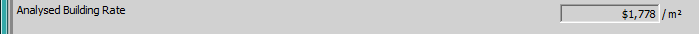## Rental Assessment

The Rental Assessment section provides the user with a set of fields to assist with the calculation of the rental return potential of the property.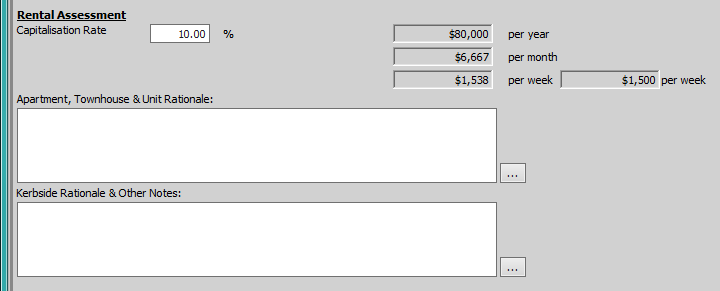### Capitalisation Rate

The Capitalisation Rate field (4) is a percentage field provided to the user to enter a rental yield percentage. This value will be saved into the RentalYield field.

The Capitalisation Rate and the Total Value field values are then used to calculate a rental income value per year, per month and per week as follows:

1. Per Year   = Total Value x ( Capitalisation Rate / 100 )
2. Per Month = Total Value x ( ( Capitalisation Rate / 100 ) / 12 )
3. Per Week  = Total Value x ( ( Capitalisation Rate / 100 ) / 52 )

The Rental Value Unfurnished value (5) entered into the job is shown in an un-editable field next to the per week calculation for easy comparison.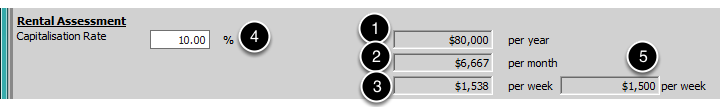### Apartment, Townhouse & Unit Rationale

The Apartment, Townhouse & Unit Rationale text field allows users to enter analysis text regarding the rental of the property. The ellipsis button [ ... ] is available beside the text field to allow the user to access the lookup list and select an option to populate the field. This value will be saved into the RentalUnitAnalysisTxt field.

This field is only visible if the Show Comment Fields in Rental Assessment Section on Analysis Tab setting is turned on.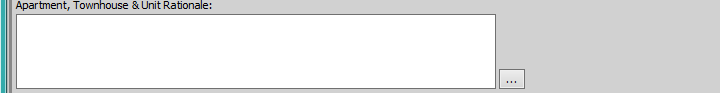### Kerbside Rationale & Other Notes

The Kerbside Rationale & Other Notes text field allows users to enter analysis text regarding the rental of the property, however this field is used if the valuation is a kerbside valuation. The ellipsis button [ ... ] is available beside the text field to allow the user to access the lookup list and select an option to populate the field. This value will be saved into the RentalKerbsideAnalysisTxt field.

This field is only visible if the Show Comment Fields in Rental Assessment Section on Analysis Tab setting is turned on.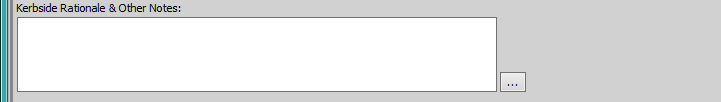## Replacement Value

This section of the Analysis tab is only visible if the Show Replacement Value Section on Analysis Tab setting is turned on.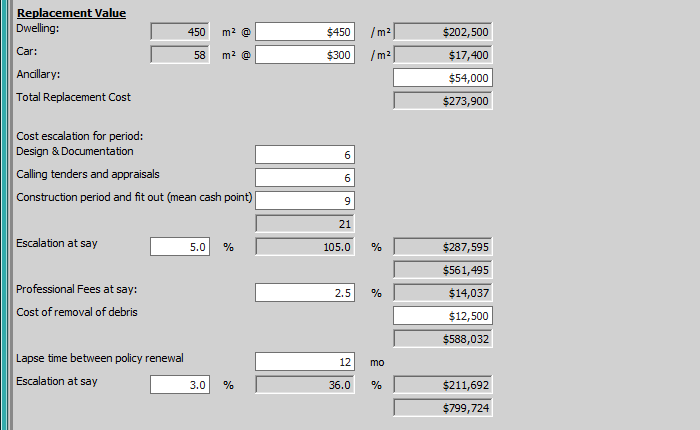### Dwelling

The Dwellings row provides the user with the following fields:

1. Area - The Area value is sourced from the Living Areas field and this field is not editable by the user. In order to change this value, the user will need to locate the Living Areas field on the Job card and update the value in that field.
2. Rate - The user can enter a building insurance rate per square metre value in this field. This value will be saved into the MainBuildingInsuranceRate field.
3. Calculated Value - The Calculated Value is the value of: Area value x Rate value.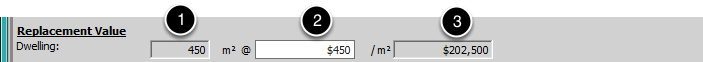### Car

The Car row provides the user with the following fields:

1. Area - The Area value is sourced from the Car Area field and this field is not editable by the user. In order to change this value, the user will need to locate the Car Area field on the Job card and update the value in that field.
2. Rate - The user can enter a car accommodation insurance rate per square metre value in this field. This value will be saved into the CarInsuranceRate field.
3. Calculated Value - The Calculated Value is the value of: Area value x Rate value.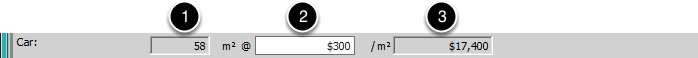### Ancillary

The Ancillary row provides the user with a field to enter the ancillary insurance value. This value will be saved into the AncillaryInsurance field.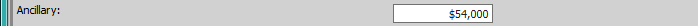### Total Replacement Cost

The Total Replacement Cost field contains the total value calculated as follows:

Dwelling Calculated Value + Car Accommodation Calculated Value + Ancillary Value = Total Replacement Cost Value

This field is not editable.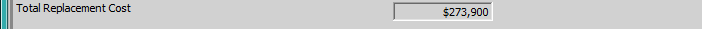### Cost Escalation for Period

The Cost Escalation For Period section provides the user with the following fields:

1. Design & Documentation - The user can enter a number of months value into this field. This value will be saved into the ReplacementEscDesignDoc field.
2. Calling tenders and appraisals - The user can enter a number of months value into this field. This value will be saved into the ReplacementEscTenders field.
3. Construction period and fit out (mean cash point) - The user can enter a number of months value into this field. The value will be saved into the ReplacementEscConstPeriod field.
4. Escalation Total - This field is not editable by the user and is used to display the total number of months of the 3 fields above.
5. Escalation at say: Monthly Escalation Percentage - This row provides the user with an editable percentage field to allow the user to enter a monthly escalation percentage value. This value will be saved into the ReplacementEscMonthlyEscalationRate field.
6. Escalation at say: Total Monthly Escalation Percentage - This field is not editable by the user and is used to display the value calculated as follows: Escalation Total x Monthly Escalation Percentage = Total Monthly Escalation Percentage
7. Replacement Cost Total - This field is not editable by the user and is used to display the value calculated as follows:  Total Replacement Cost x ( ( Total Monthly Escalation Percentage / 100 ) ) = Replacement Cost Sub Total
8. Replacement Cost Sub Total 1 - This field is not editable by the user and is used to display the sub total value calculated as follows:  Total Replacement Cost x ( 1 + ( Total Monthly Escalation Percentage / 100 ) ) = Replacement Cost Sub Total 1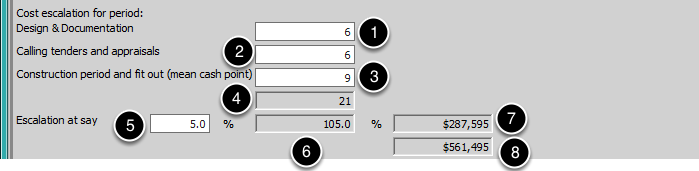### Professional Fees

The Professional Fees section provides the user with the following fields:

1. Professional Fees Rate - The user is presented with an editable percentage field to allow the user to enter a professional fee rate value. This value will be saved into the ReplacementProfFeesRate field.
2. Total Professional Fees - This field is not editable by the user and is used to display the value calculated as follows:

Replacement Cost Sub Total 1 x ( Professional Fees Rate / 100 ) = Total Professional Fees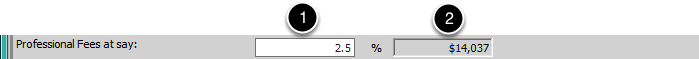### Cost Of Removal of Debris

The Cost of Removal of Debris section provides the user with the following fields:

1. Debris Removal Fee - The user is presented with an editable currency field to allow the user to enter a fee for the debris removal value. This value will be saved into the ReplacementDebrisRemovalFee field.
2. Replacement Cost Sub Total 2 - This field is not editable by the user and is used to display the running sub total value of the replacement cost value calculated as follows:

Replacement Cost Sub Total 1 + Total Professional Fees + Debris Removal Fee = Replacement Cost Sub Total 2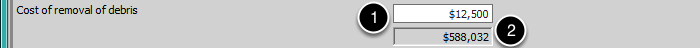### Lapse Time Between Policy Renewal

The Lapse Time Between Policy Renewal section provides the user with the following fields:

1. Lapse Time Between Policy Renewal - The user can enter a number of months value into this field. This value will be saved into the ReplacementEscRenewalPeriod field.
2. Escalation at say: Monthly Escalation Rate Percentage - This row provides the user with an editable percentage field to allow the user to enter a monthly escalation rate percentage value. This value will be saved into the ReplacementEscCostRenewalRate field.
3. Escalation at say: Total Monthly Escalation Percentage - This field is not editable by the user and is used to display the value calculated as follows: Monthly Escalation Rate Percentage x Lapse Time Between Policy Renewal = Total Monthly Escalation Percentage
4. Escalation Renewal Cost Total - This field is not editable by the user and is used to display the value calculated as follows:  Replacement Cost Sub Total 2 x ( ( Total Monthly Escalation Percentage / 100 ) ) = Escalation Renewal Cost Total
5. Replacement Cost Total - This field is not editable by the user and is used to display the total replacement cost value calculated as follows:

Replacement Cost Sub Total 3 x ( 1 + ( Total Monthly Escalation Percentage / 100 ) ) = Replacement Cost Total